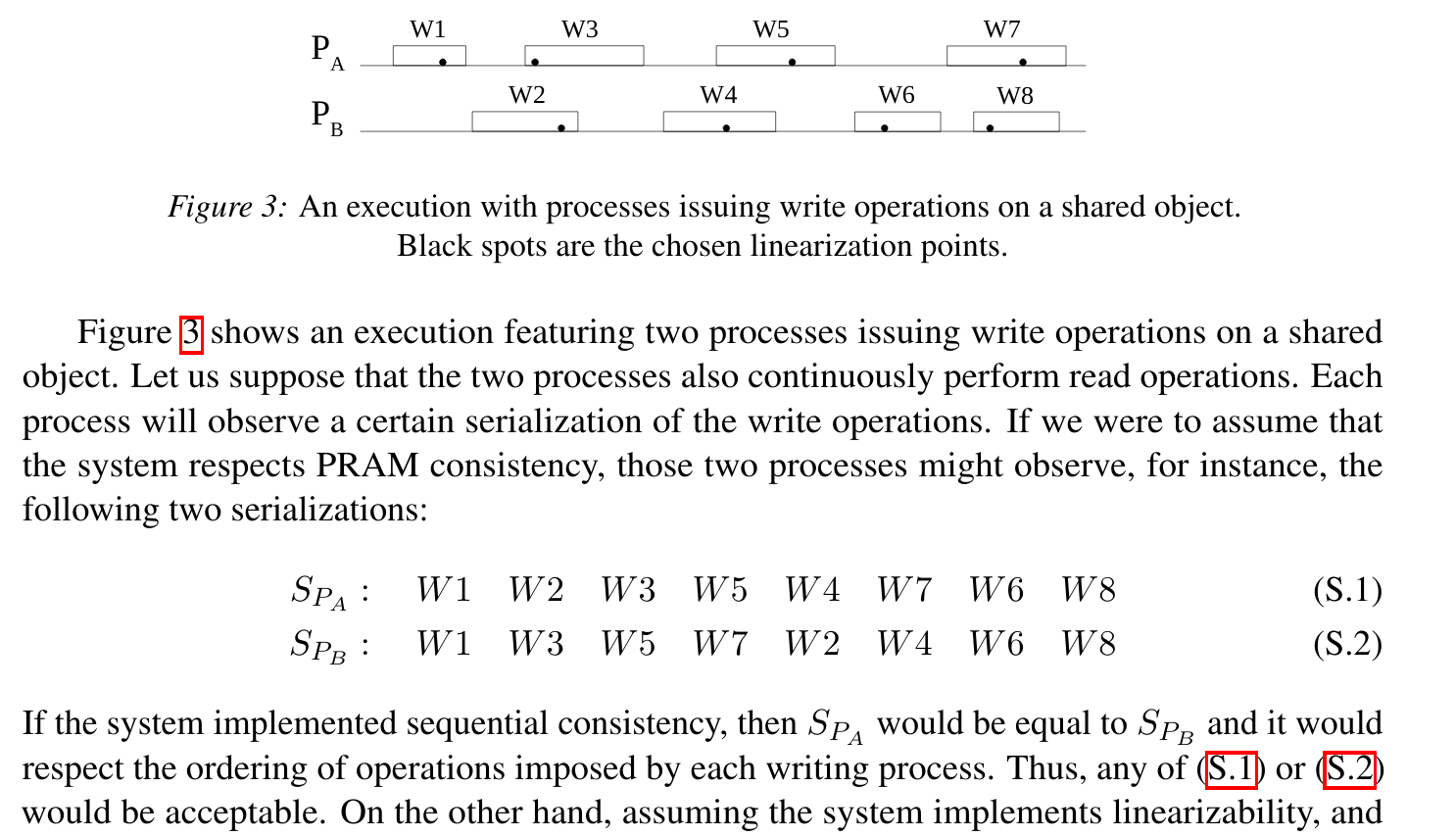Sequential Consistency Described by Viotti and Vukolic

A friend and I were discussing how sequential consistency works, especially in regards to the Viotti and Vukolic paper. I figured it would be useful to write down how the paper lays out sequential consistency.

(EDIT: My friend also wrote up his thoughts: https://www.ldelossa.is/blog/sequential-consistency-in-practice/)

Jepsen describes sequential consistency as:

Sequential consistency is a strong safety property for concurrent systems. Informally, sequential consistency implies that operations appear to take place in some total order, and that that order is consistent with the order of operations on each individual process.

Which gives a pretty good theoretical description. Viotti and Vukolic (page 12) go further, giving a concrete example:Ignoring the information about lineraization and breaking this down:

• There are two processes, each issuing writes.
• Process A ($$P_A$$) issues writes in the order of W1, W3, W5, and W7.
• Process B ($$P_B$$)issues writes in the order of W2, W4, W6, and W8.
• To have sequential consistency, these writes can be interleaved in any way, as long as the writes preserve the same order.

Viotti and Vukolic give us two examples:

• $$S_{p_a}$$: W1 W2 W3 W5 W4 W7 W6 W8
• $$S_{p_b}$$: W1 W3 W5 W7 W2 W4 W6 W8

We can then use the givens to validate the two examples are, in fact, sequentially consistent.

1. Example 1

To validate $$S_{p_a}$$, we can look at the writes for each process.

For $$P_A$$ writes in $$S_{p_a}$$ (process A writes in bold):

• W1 W2 W3 W5 W4 W7 W6 W8

We can see that the set of {W1, W3, W5, W7} does indeed match the correct order of writes that Process A sees.

For $$P_B$$ writes in $$S_{p_a}$$ (process B writes in italics):

• W1 W2 W3 W5 W4 W7 W6 W8

We can again see that the set of {W2, W4, W6, W8} matches the writes in Process B.

2. Example 2

The second example is slightly different, it has all the writes of process A first, followed by all the writes of Process B.

Using bold to denote process A writes and italics to denote process B writes:

$$S_{p_b}$$: W1 W3 W5 W7 W2 W4 W6 W8

Looking at each write, observed from the point of each of the processes, it's clear that $$S_{p_b}$$ follows the order the writes came in (as required by PRAM). This means that $$S_{p_b}$$ is still sequentially consistent (although it is not linearizable).

3. Other Examples

The writes defined by $$P_A$$ and $$P_B$$ can be arranged in ways beyond $$S_{p_a}$$ and $$S_{p_b}$$ to follow sequential consistency. For example:

$$S_{p_c}$$: W2 W4 W6 W8 W1 W3 W5 W7

Here, $$S_{p_c}$$ is the inverse of $$S_{p_b}$$, where the writes for $$P_B$$ happen first, followed by $$P_A$$. Because total order is still followed (i.e. W7 is always follows W5, W8 always follows W6), this is still sequentially consistent.

Another example could be:

$$S_{p_d}$$: W2 W1 W3 W5 W7 W4 W6 W8

This sequence is still sequentially consistent, because a total order is still respected. Even though all the writes for $$P_A$$ are interleaved between W2 and W4, the ordering from each process is still followed.

To put it another way, sequential consistency ties together the writes on a single process to the order. Each process's writes can be interleaved with the writes from other processes, but they cannot be rearranged.

Posted: 2020-07-16
Filed Under: computers